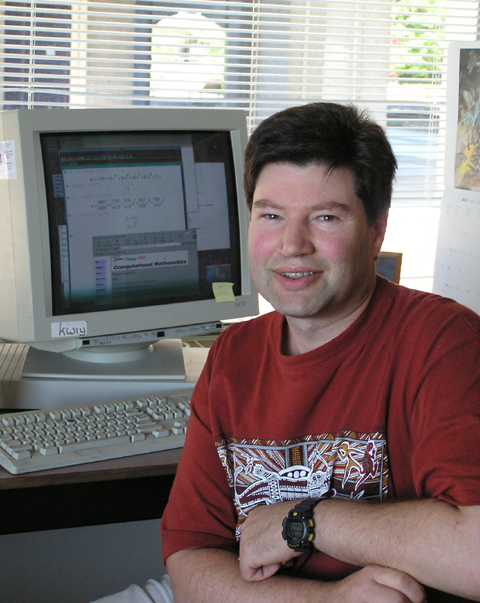Teaching and Doing Mathematics in Maple 10

With Michael Monagan, Vancouver, August 14th - 18th, 2006.Dates: August 14th - 18th, 2006. Venue: Room K9509, Shrum Science Centre, Simon Fraser University. Instructor: Michael Monagan: mmonagan AT cecm DOT sfu DOT ca Course Brochure in Microsoft Word format (.doc) Course Brochure in Adobe pdf format (.pdf) The Maple worksheets and support materials used in the course can be downloaded. The files MAPLE06.zip contains a zip archive of all the files that you can download. The file FILES.txt contains a list of the files.

Course Outline

Participants may attend any 2 to 5 days.

Monday August 14th: Introduction to Maple

Using Maple as a calculator. Graphing curves and functions and creating simple animations. Solving equations exactly and numerically. Input of mathematical formulae. Expanding and factoring polynomials. Simplifying formulae. The Maple GUI: saving Maple worksheets, exporting worksheets to HTML. Creating a document with text, mathematical formulae, and plots in Maple.

Tuesday August 15th: Calculus, 3D Graphics and Statistics

Taylor series and limits. Indefinite and definite integration in Maple. Animations of convergence of Riemann sums and series. Graphing curves and surfaces in 3 dimensions. Visuals of partial derivatives and tangent planes. Solving systems of equations and finding critical points. Computing multivariate Taylor series. Looping constructs in Maple and manipulating data sets.

Wednesday August 16th: Linear Algebra and Preparing Documents

Vectors, matrices and linear systems in Maple. Visualization of linear systems and eigenvectors for teaching. Least squares approximation. About numerical linear algebra facilities available in Maple. Solving non-linear systems of polynomial equations, factoring polynomials and Groebner bases. More on the Maple GUI. Creating teaching and presentation materials in Maple worksheets. Exporting Maple worksheets to RTF, LaTeX and HTML.

Thursday August 17th: Differential Equations and Animations

Examples of first and second order equations and first order systems; their and their exact and numerical solution in Maple. Visualization of systems using phase-portrait plots and animation. An analysis of the local stability of the stationary points of the modified Lotka Volterra system. Creating your own 2D and 3D graphics images. Assembling your own animations.

Friday August 18th: Programming in Maple

We will introduce loop constructs, Maple procedures and modules (packages) using the following examples. The Euclidean algorithm - programming with long integers. A simple 1D cellular automaton - programming with arrays. Creating fractal images - programming with graphics objects. Symbolic differentiation of a formula - programming with formulae. A package of routines for drawing electronic components - creating a module.